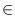Next: Lossless Compression Up: Priority Queues Previous: Priority Queues ( p_queue   Contents   Index

# Bounded Priority Queues ( b_priority_queue )

Definition

An instance Q of the parameterized data type b_priority_queue<I> is a collection of items (type bpqitem). Every item contains a priority from a fixed interval [a..b] of integers (type int) and an information from an arbitrary type I. The number of items in Q is called the size of Q. If Q has size zero it is called the empty priority queue. We use < p, i > to denote a b_pq_item with priority p[a..b] and information i.
Remark: Iteration over the elements of Q using iteration macros such as forall is not supported.

#include < LEDA/core/b_prio.h >

Creation

 b_priority_queue Q(int a, int b) creates an instance Q of type b_priority_queue with key type [a..b] and initializes it with the empty priority queue.

Operations

 b_pq_item Q.insert(int key, const I& inf) adds a new item < key, inf > to Q and returns it. Precondition key[a..b] void Q.decrease_key(b_pq_item it, int newkey) makes newkey the new priority of item it. Precondition it is an item in Q, newkey[a..b] and newkey is not larger than prio(it). void Q.del_item(b_pq_item x) deletes item it from Q. Precondition it is an item in Q. int Q.prio(b_pq_item x) returns the priority of item i. Precondition it is an item in Q. const I& Q.inf(b_pq_item x) returns the information of item i. Precondition it is an item in Q. b_pq_item Q.find_min() returns an item with minimal priority (nil if Q is empty). I Q.del_min() deletes the item it = Q.find_min() from Q and returns the information of it. Precondition Q is not empty. void Q.clear() makes Q the empty bounded prioriy queue. int Q.size() returns the size of Q. bool Q.empty() returns true if Q is empty, false otherwise. int Q.lower_bound() returns the lower bound of the priority interval [a..b]. int Q.upper_bound() returns the upper bound of the priority intervall [a..b].

Implementation

Bounded priority queues are implemented by arrays of linear lists. Operations insert, find_min, del_item, decrease_key, key, inf, and empty take time O(1), del_min (= del_item for the minimal element) takes time O(d ), where d is the distance of the minimal element to the next bigger element in the queue (= O(b - a) in the worst case). clear takes time O(b - a + n) and the space requirement is O(b - a + n), where n is the current size of the queue.Next: Lossless Compression Up: Priority Queues Previous: Priority Queues ( p_queue   Contents   Index